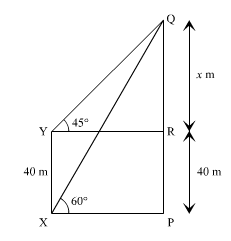Courses
Courses for Kids
Free study material
Free LIVE classes
MoreLIVE
Join Vedantu’s FREE Mastercalss

# The angle of elevation of the top Q of a vertical tower PQ from a point X on the ground is ${60^ \circ }$. From a point Y, 40m vertically above X, the angle of elevation of the top Q of the tower is ${45^ \circ }$. Find the height of the tower PQ and the distance PX. (Use $\sqrt 3 = 1.73$)Verified
261.6k+ viewsHint: In this question, we are supposed to find the length of tower and distance between point P and point X. We are going to consider the $\Delta YRQ$ and find the value of YR and XP from there and similarly, find the value of PX from it.
In $\Delta YRQ$, we have,
$\tan {45^ \circ } = \dfrac{{QR}}{{YR}}$
$1 = \dfrac{x}{{YR}}$
$\Rightarrow YR = x$
Or $XP = x\left[ {As\,YR = XP} \right]$ …..(1)
Now, In $\Delta XPQ$ , we have,
$\tan {60^ \circ } = \dfrac{{PQ}}{{PX}}$
$\sqrt 3 = \dfrac{{x + 40}}{x}$ [Using (1)]
$\sqrt 3 x = x + 40$
$XQ = 109.3m$
$x = \dfrac{{40}}{{\sqrt 3 - 1}}$
On rationalising the denominator, we get,
$x = \dfrac{{40}}{{\sqrt 3 - 1}} \times \dfrac{{\sqrt 3 + 1}}{{\sqrt 3 + 1}}$
$x = \dfrac{{40\sqrt 3 + 1}}{{3 - 1}}$
$x = \dfrac{{40\sqrt 3 + 1}}{2}$
$x = 20\left( {\sqrt 3 + 1} \right) = 54.64m$
So, height of the tower, PQ= x+40 = 54.64 + 40 = 94.64m
Now in $\Delta XPQ$, we have,
$\sin {60^ \circ } = \dfrac{{PQ}}{{XQ}}$
$\dfrac{{\sqrt 3 }}{2} = \dfrac{{94.64}}{{XQ}}$
$XQ = \dfrac{{94.64 \times 2}}{{\sqrt 3 }}$
$XQ = \dfrac{{94.64 \times 2 \times \sqrt 3 }}{{\sqrt 3 \times \sqrt 3 }}$
Note: In these questions, make sure you take the correct trigonometric angle.
Answer = $XQ = 109.3m$

Last updated date: 19th Sep 2023
Total views: 261.6k
Views today: 5.61k